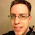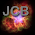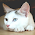## Friday, November 11, 2011

### Quaternion Math: Getting local axis vectors from a quaternion

If your game uses the standard coordinate system of X representing left/right vector, Y representing up/down vector, and Z representing forward/backward vector, then this following method should allow you to convert a quaternion into a vector that represents 'forward', 'up', and 'right'.

I've found these extremely useful, as this is much more efficient than converting the quaternion into a matrix just so you can extract the column/row you need from the matrix for the vector you want.

```Vector3 Quaternion::GetForwardVector() const
{
return Vector3( 2 * (x * z + w * y),
2 * (y * x - w * x),
1 - 2 * (x * x + y * y));
}

Vector3 Quaternion::GetUpVector() const
{
return Vector3( 2 * (x * y - w * z),
1 - 2 * (x * x + z * z),
2 * (y * z + w * x));
}

Vector3 Quaternion::GetRightVector() const
{
return Vector3( 1 - 2 * (y * y + z * z),
2 * (x * y + w * z),
2 * (x * z - w * y));
}
```

1.Thank you so much! This is not (and very much should be) built in to Unity's quaternions.

1.Also not built into Gamebryo. Was helpful having these on my last game project (since Gamebryo lacked this) so I thought it would be worth posting in the case that other math libraries were lacking it as well.

2.Hi Nic, your post really helped me out on a project for school. I'll be sure to refer classmates to your website in the future.

I just started blogging myself and I hope I have some topics that could be of help to you:
www.mindbreakinggames.com

3.Thanks, not sure why these simple functions are so hard to track down. BUT there's an error in GetForwardVector(). The Y value is incorrect. It should be:

Vector3 Quaternion::GetForwardVector() const
{
return Vector3( 2 * (x * z + w * y),
2 * (y * z - w * x),
1 - 2 * (x * x + y * y));
}

4.Very useful article. Many Thanks.

I have converted this in to C# for use with XNA and posted an article on my blog: http://blog.diabolicalgame.co.uk/2013/06/quaternion-axes.html

To match the XNA Matrix forward axis I had to change the forward vector for the quaternion to:

return Vector3( -2 * (x * z + w * y),
-2 * (y * z - w * x),
-1 + 2 * (x * x + y * y));
}

5.It is dangerous to include such a helper method because it assumes the users cordinate system. It also is not doing any less math than the following:
var posy = Vector3.Transform(new Vector3(0,1,0), quat);

6.Hey! Could you provide where you get it formula? References?